# Python 让书法作品和 PIL 库来一场美丽的邂逅## 2. 遮罩图片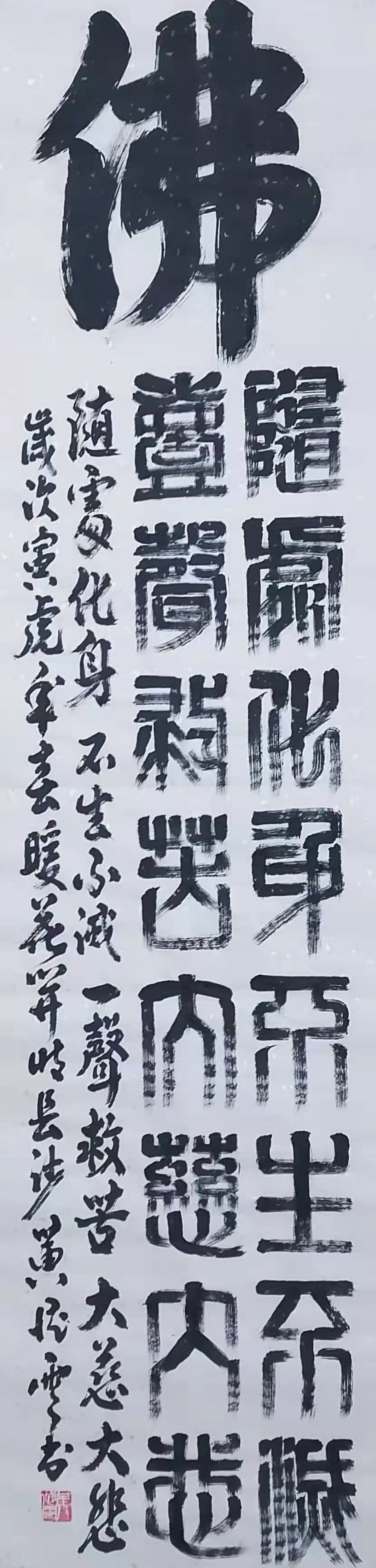### 2.1 使用 Image 模块的裁剪方法

``````from PIL import Image
# 打开原图片
fo_img = Image.open("fo.jpg")
# 因后续要使用此图片做遮罩，需要透明通道，所以要转换成 RGBA 模式
fo_img = fo_img.convert("RGBA")
# 获取图片本身大小
w, h = fo_img.size
# h/4-55 完全是试出来的偏差值
fo_img = fo_img.crop((0, 0, w, h / 4 - 55))
fo_img.show()
``````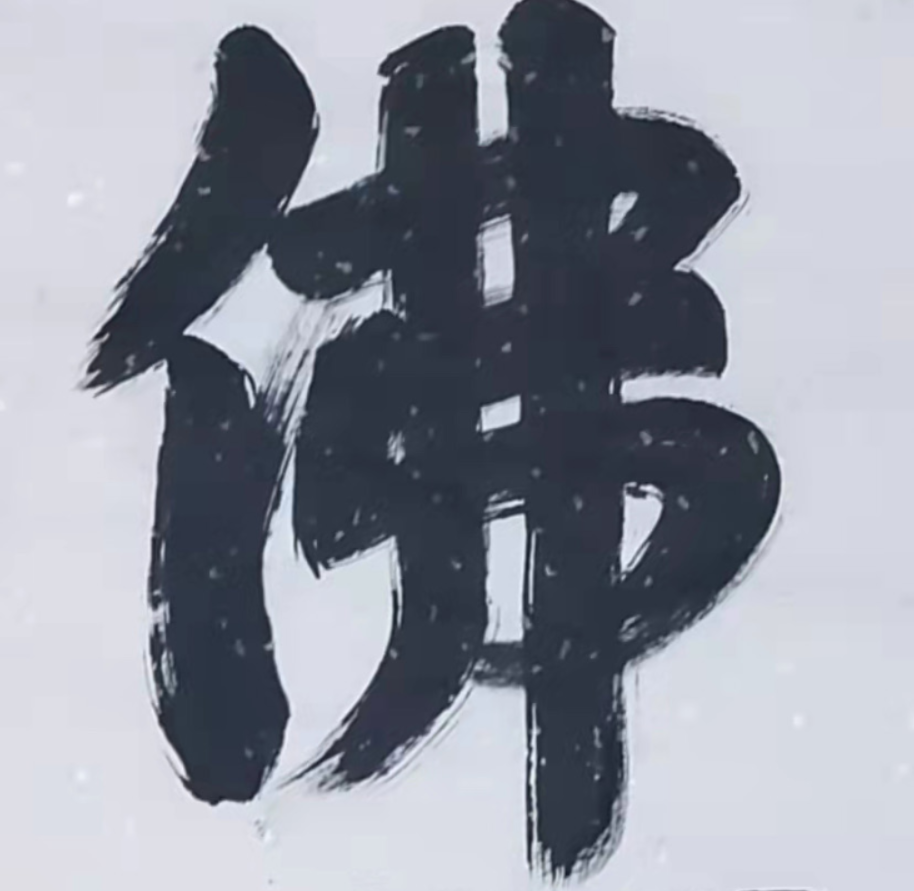### 2.2 一个像素点一个像素点的裁剪

Tip： 当使用一张图片做遮罩时，图片的 a 通道值为 0 的地方，被遮罩图片所遮住的图片会变成透明。a 通道为 255 的地方，表示完全不透明，从 0 到 255 之间由透明逐渐梯度变成不透明。

``````from PIL import Image
fo_img = Image.open("fo.jpg")
# 先转换成 RGBA 模式
fo_img = fo_img.convert("RGBA")
# 获取图片本身大小
w, h = fo_img.size
# 创建一张空白的新图片，大小和要裁剪的佛字图片一样大小
fo_only_img = Image.new(fo_img.mode, (w, int(h / 4) - 55))
w, h = fo_only_img.size
for i in range(w):
for j in range(h):
# 获取每一像素点的颜色分量
r, g, b, a = fo_img.getpixel((i, j))
# 把白色区域的 a 值修改为 0 ，白色区域的R，G，B值相近
if r > 180 or g>180 or b>180:
a = 0
# 为新图片指定新的颜色模式
fo_only_img.putpixel((i, j), (r, g, b, a))
fo_only_img.show()
``````

• 第一种方案提取后，还是需要再修改每一个像素点的透明信息。

• 第 2 种方案一步到位。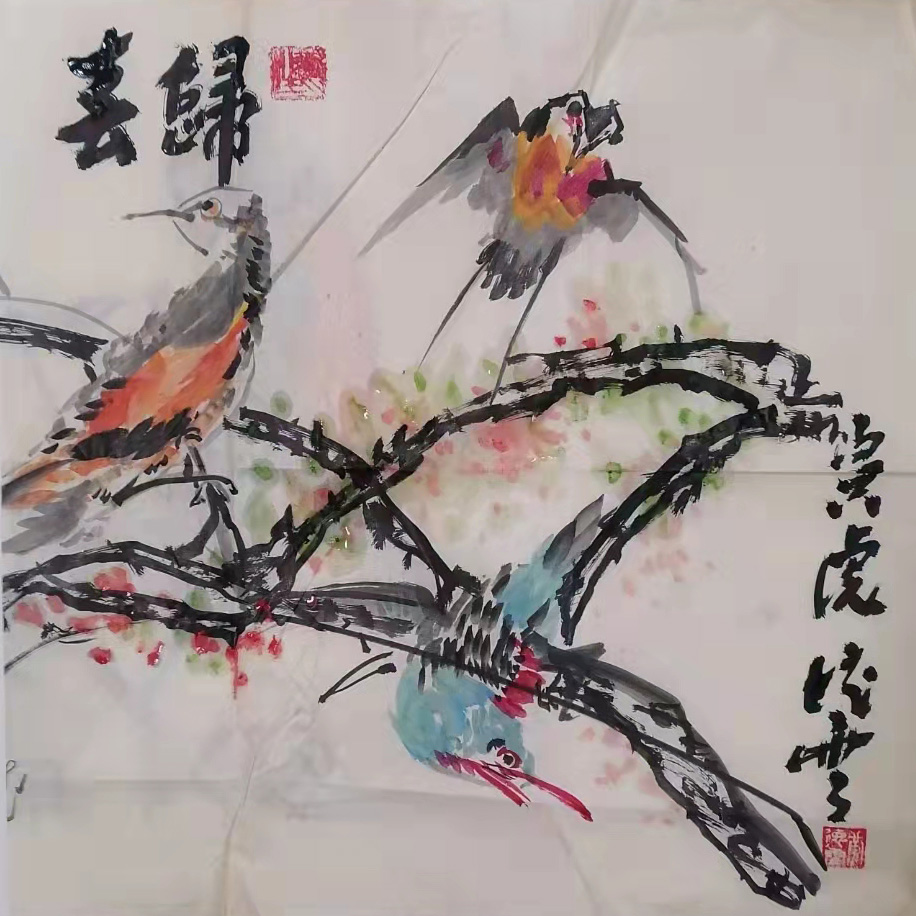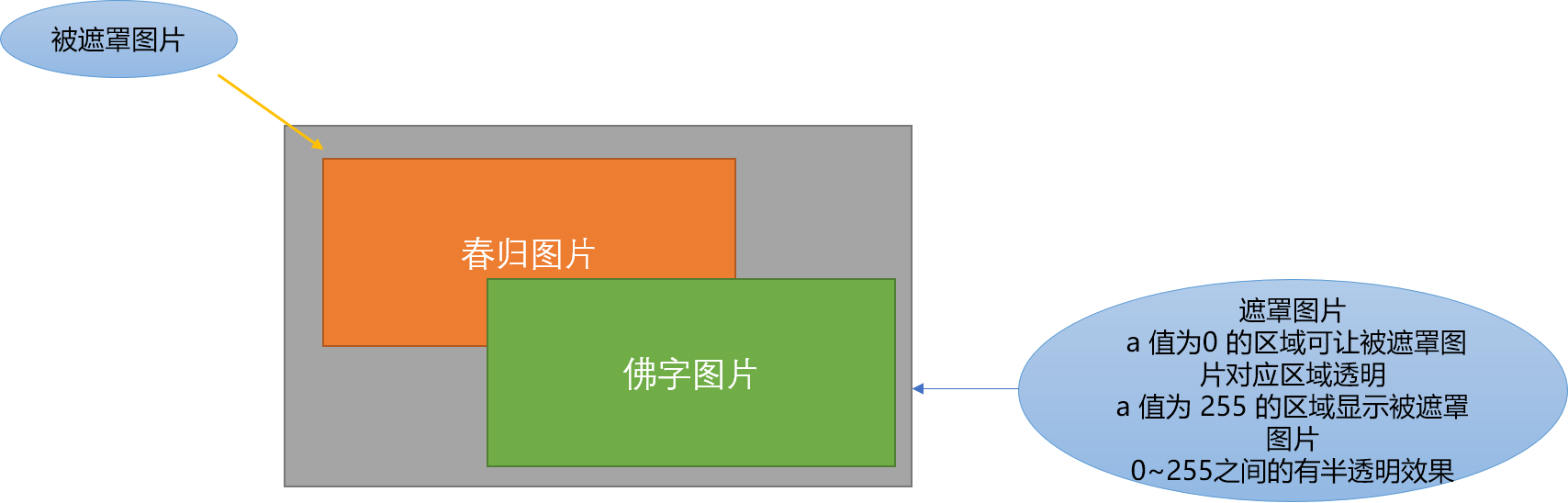``````from PIL import Image
fo_img = Image.open("fo.jpg")
# 先转换成 RGBA 模式
fo_img = fo_img.convert("RGBA")
# 获取图片本身大小
w, h = fo_img.size
# 创建一张空白的新图片，大小和要裁剪的佛字图片一样大小
fo_only_img = Image.new(fo_img.mode, (w, int(h / 4) - 55))
w, h = fo_only_img.size
for i in range(w):
for j in range(h):
# 获取每一像素点的颜色分量
r, g, b, a = fo_img.getpixel((i, j))
# 把白色区域的 a 值修改为 0
if r > 180:
a = 0
# 为新图片指定新的颜色模式
fo_only_img.putpixel((i, j), (r, g, b, a))
# 开始准备做遮罩效果之前，打开被遮罩图
chun_gui_img = Image.open("chungui.jpg")
# 修改 chun_gui_img 图片和遮罩图片一样大小
chun_gui_img = chun_gui_img.resize(fo_only_img.size)
# 创建一张新的、空白的、纯白色的背景图片
new_img = Image.new("RGBA", chun_gui_img.size)
# 开始粘贴
new_img.show()
``````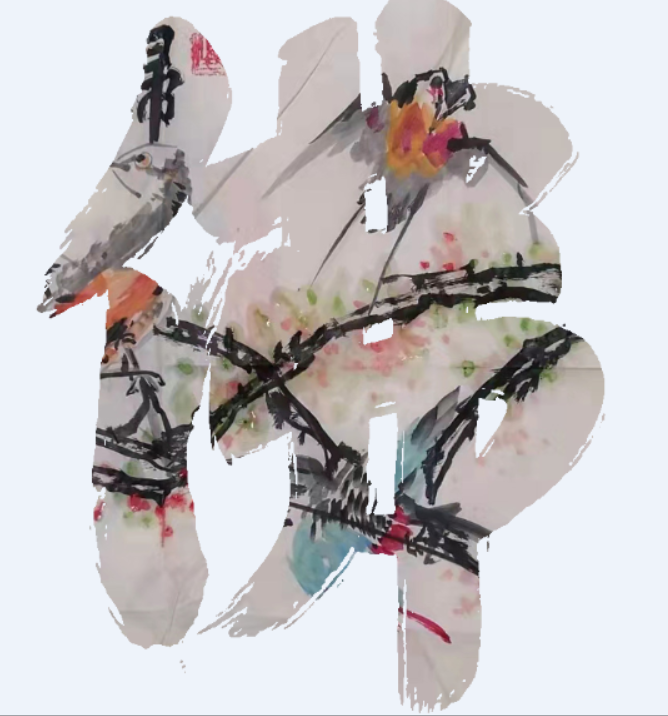1. 反转效果

前面是把佛字图片的白色区域的 a 值设定为 0，则白色区域所对应的春归图片会变成透明。现在反过来，把文字区域的 a 值设为 0。就可以看到和上图相反的一个效果。

``````from PIL import Image
import random

fo_img = Image.open("fo.jpg")
# 先转换成 RGBA 模式
fo_img = fo_img.convert("RGBA")
# 获取图片本身大小
w, h = fo_img.size
# 创建一张空白的新图片，大小和要裁剪的佛字图片一样大小
fo_only_img = Image.new(fo_img.mode, (w, int(h / 4) - 55))
w, h = fo_only_img.size
# 步长值
step = 1
step1 = 1
for i in range(0, w, 1):
for j in range(h):
# 获取每一像素点的颜色分量
r, g, b, a = fo_img.getpixel((i, j))
# 文字区域的 a 值设置为 0
if r < 80:
a = 0
# 为新图片指定新的颜色模式
fo_only_img.putpixel((i, j), (r, g, b, a))
# 开始准备做遮罩效果之前，先打开底图
chun_gui_img = Image.open("chungui.jpg")
# 修改 chun_gui_img 图片和遮罩图片一样大小
chun_gui_img = chun_gui_img.resize(fo_only_img.size)
# 创建一张新的图片
new_img = Image.new("RGBA", chun_gui_img.size,(100,200,80))
# 开始粘贴
new_img.show()

``````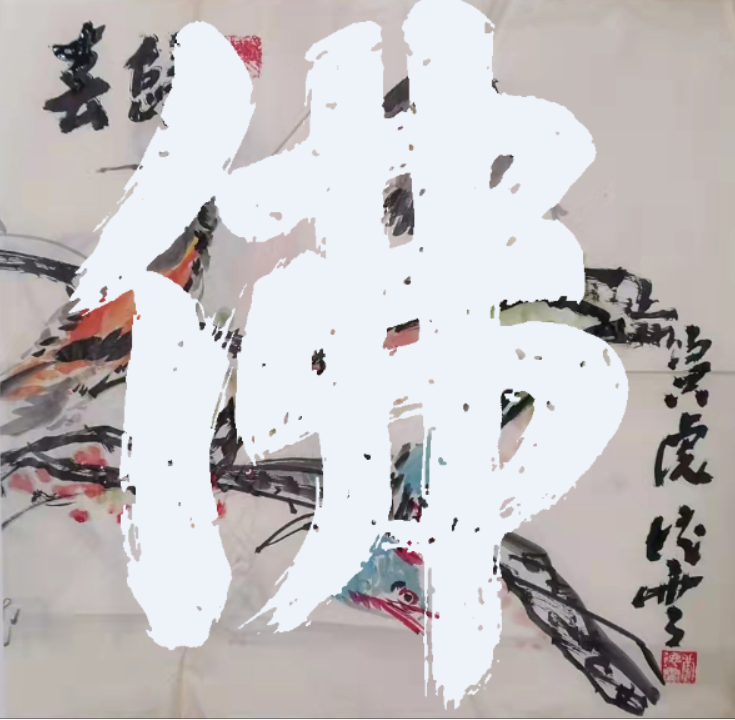``````new_img = Image.new("RGBA", chun_gui_img.size,ImageColor.getrgb("gold"))
``````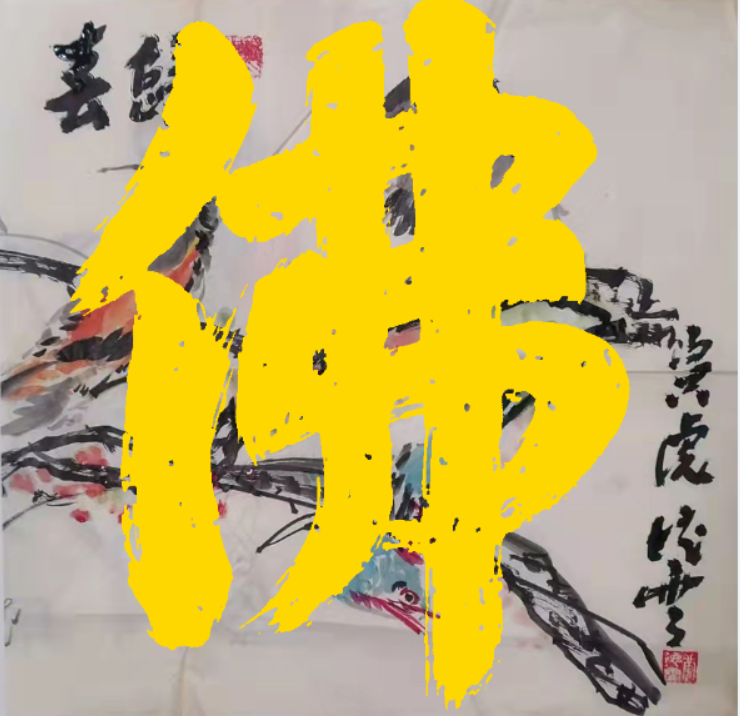1. 颗粒效果

``````from PIL import Image
import random
fo_img = Image.open("fo.jpg")
# 先转换成 RGBA 模式
fo_img = fo_img.convert("RGBA")
# 获取图片本身大小
w, h = fo_img.size
# 创建一张空白的新图片，大小和要裁剪的佛字图片一样大小
fo_only_img = Image.new(fo_img.mode, (w, int(h / 4) - 55))
w, h = fo_only_img.size
print(w)
# 步长值
step = 1
for i in range(0, w, 1):
for j in range(h):
# 获取每一像素点的颜色分量
r, g, b, a = fo_img.getpixel((i, j))
if r > 180:
a = 0
elif r < 100:
# 随机
a -= random.randint(0, 255)
# 为新图片指定新的颜色模式
fo_only_img.putpixel((i, j), (r, g, b, a))
# 开始准备做遮罩效果之前，先打开底图
chun_gui_img = Image.open("chungui.jpg")
# 修改 chun_gui_img 图片和遮罩图片一样大小
chun_gui_img = chun_gui_img.resize(fo_only_img.size)
# 创建一张新的图片
new_img = Image.new("RGBA", chun_gui_img.size)
# 开始粘贴
new_img.show()

``````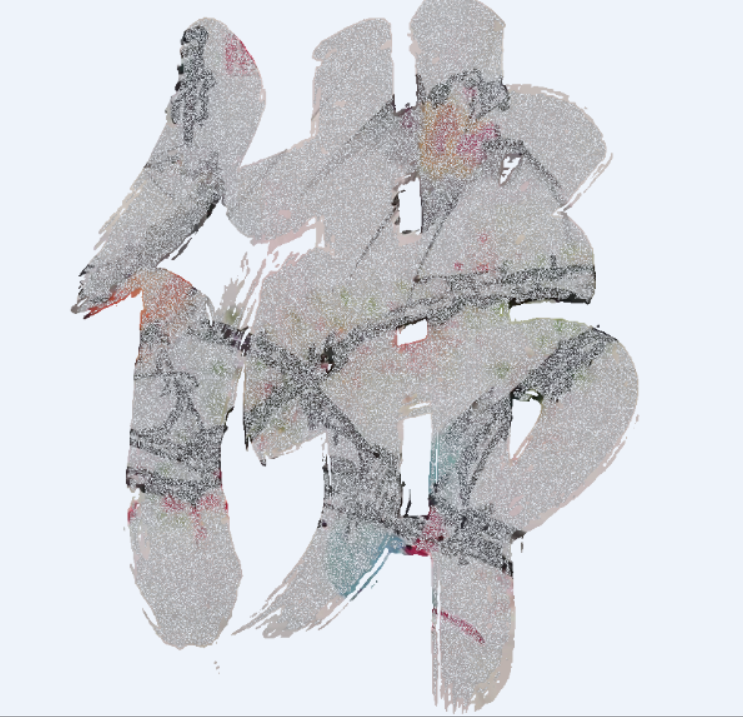## 3. 字符串图片

``````from PIL import Image
import random
fo_img = Image.open("fo.jpg")
# 先转换成 RGBA 模式
fo_img = fo_img.convert("RGBA")
# 获取图片本身大小
w, h = fo_img.size
# 创建一张空白的新图片，大小和要裁剪的佛字图片一样大小
fo_only_img = Image.new(fo_img.mode, (w, int(h / 4) - 55))
w, h = fo_only_img.size
# 步长值
old_rgb = None
s = ''
for i in range(w):
for j in range(h):
# 获取每一像素点的颜色分量
r, g, b, a = fo_img.getpixel((i, j))
fo_only_img.putpixel((i, j), (r, g, b, a))

fo_only_img = fo_only_img.resize((300, 300))
w, h = fo_only_img.size
for i in range(h):
for j in range(w):
r, g, b, a = fo_only_img.getpixel((j, i))
if r > 100:
s += "仁"
else:
s += '佛'
s += "\n"
with open("d:/fo.txt", 'w') as f:
f.write(s)
``````### 4. 总结